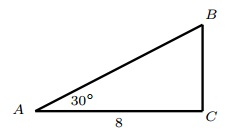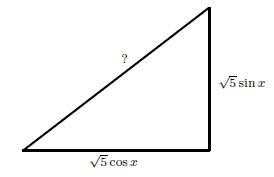# Right Triangle Questions

Multiple choice questions right triangle problems related to trigonometry with answers at the bottom of the page.

### Question 1

What is the measure of angle A in the right triangle below?a) 17�
b) 27�
c) 17�
d) 90�

### Question 2

What is the value of the side x in the right triangle below?a) 1
b) 9
c) 20
d) 3

### Question 3

In a right triangle, the measure of one of the angles is 49� and the hypotenuse has a length of 50 cm. Which of the following is the nearest approximation to the length, in cm, of the leg opposite to this angle?
a) 32.8
b) 57.5
c) 37.7
d) 30.3

### Question 4

In the right triangle ABC below, angle A measures 30� and the length of AC is 8 units. Find the length of BCa) 8 / √ 3
b) 4 / √ 3
c) 4
d) 8

### Question 5

In the right triangle below, what is sin α?a) 13 / 9
b) 9 / 13
c) 13 √10 / 50
d) 13 / 24

### Question 6

Find the length of AC in the right triangle below.a) 9
b) 9 √2
c) 18 √2
d) 18

### Question 7

Find the length of the hypotenuse in the right triangle below where x is a real number.a) 5
b) 10
c) 25
d) √ 5

### Question 8

Find the area of a square whose diagonal is 40 meters.
a) 80 m
2
b) 800 m
2
c) 1600 m
2
d) 40 m
2

### Question 9

In the figure below BC is perpendicular to AD, CD = 8, the measure of angle D is 60� and the measure of angle A is 45�. Find the length of ABa) 8 √6
b) 8 √3
c) 8 √2
d) 8

### Question 10

What is the length of AB in the figure below.a) 12 √2
b) 12
c) 12 √3
d) 12 √6

### Question 11

In the figure below, find cosθ.a) 3 / 5
b) 4 / 5
c) 1 / 5
d) 2 / 5

### Question 12

In the triangle below, m = ?a) 5
b) 10 √2
c) 20 √2
d) 5 √2

1. b)
2. d)
3. c)
4. a)
5. c)
6. b)
7. d)
8. b)
9. a)
10. c)
11. b)
12. d)